# Texas Go Math Grade 5 Lesson 1.6 Answer Key Estimate Decimals Sums and Differences

Refer to our Texas Go Math Grade 5 Answer Key Pdf to score good marks in the exams. Test yourself by practicing the problems from Texas Go Math Grade 5 Lesson 1.6 Answer Key Estimate Decimals Sums and Differences.

## Texas Go Math Grade 5 Lesson 1.6 Answer Key Estimate Decimals Sums and Differences

Unlock the Problem

A singer is recording a CD. The lengths of the three songs are 3.4 minutes, 2.78 minutes, and 4.19 minutes. About how much recording time will be on the CD?

Use rounding to estimate.

Round to the nearest whole number. Then add.Remember
To round a number, determine the place to which you want to round.
. If the digit to the right is less than 5, the digit in the rounding place stays the same.
. If the digit to the right is 5 or greater, the digit in the rounding place increases by 1.
So,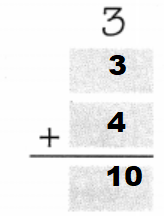So,
There will be about 10 minutes of recording time on the CD.

Try This!

Use rounding to estimate.

A.
Round to the nearest whole dollar. Then subtract.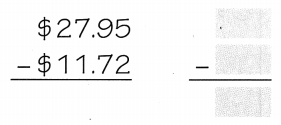To the nearest dollar, $27.95 –$11.72 is about _________.
The given numbers are: 27.95 and 11.72
Now,
When we round off the given numbers to the nearest whole numbers,
The numbers will become: 28 and 12
Now,Hence, from the above,
We can conclude that
To the nearest dollar, the subtraction of the given values is about $16 B. Round to the nearest ten dollars. Then subtract.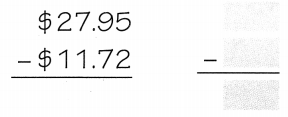To the nearest ten dollar,$27.95 – $11.72 is about _________. Answer: The given numbers are: 27.95 and 11.72 Now, When we round off the given numbers to the nearest tenths, The numbers will become: 28.0 and 11.7 So,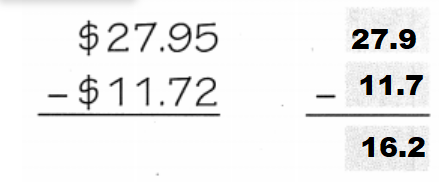Hence, from the above, We can conclude that The value of the given numbers when rounded off to the nearest tenth is about$16.2

Do you want an overestimate or an underestimate when you estimate the total cost of items you want to buy? Explain.
Easy all you have to do it estimate.
Example:
$11.62 +$7.86 = $19.48$11.62 rounds to 12 and $7.80 rounds to$8.00
So,
The round to the total would be $20 so this would be an over estimation Use Benchmarks Benchmarks are familiar numbers used as points of reference. You can use the benchmarks 0, 0.25, 0.50, 0.75, and 1 to estimate decimal sums and differences. Example Use benchmarks to estimate. 0.76 – 0.22 Locate and graph a point on the number line for each decimal. Identify which benchmark each decimal is closer to.So, 0.76 – 0.22 is about ____________. Answer: We know that, “Benchmarks” are the numbers that act as a point of reference and the benchmark numbers are: 0, 0.25, 0.50, and 0.75 Now,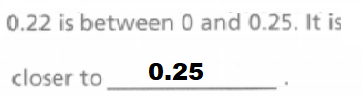So, The difference between 0.76 and 0.22 with the reference to the benchmarks will be: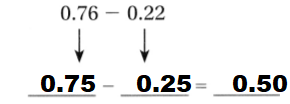Hence, from the above, We can conclude that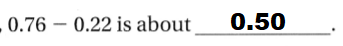Math Talk Mathematical Processes Use the Example to explain how using rounding or benchmarks to estimate a decimal difference can give you different answers. Answer: To estimate means to make a rough guess or calculation. To round means to simplify a known number by scaling it slightly up or down. Rounding is a type of estimation. Both methods can help you make educated approximations and can be used in everyday life for tasks related to money, time, or distance. A benchmark helps a student estimate the general number a fraction or decimal number is. For example, a student can quickly learn that the fraction $$\frac{1}{2}$$ means a half, 0.50, or 50 percent because of intuition. Share and Show Use rounding to estimate. Question 1.Answer: The given numbers are: 2.34, 1.9, and 5.23 So,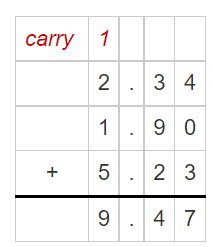Hence, from the above, We can conclude that The rounded value for the given addition of given numbers is: 9.5 Question 2.Answer: The given numbers are: 10.39, 4.28 So,Hence, from the above, We can conclude that The rounded value for the subtraction of given numbers is: 6.1 Question 3.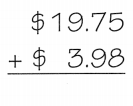Answer: The given numbers are: 19.75, 3.98 So,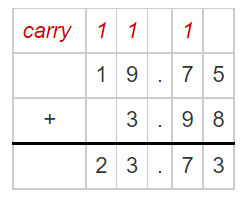Hence, from the above, We can conclude that The rounded value for the addition of given numbers is:$23.7

Use benchmarks to estimate.

Question 4.The given numbers are: 0.34, 0.1, and 0.25
Now,
We know that,
The benchmarks are: 0, 0.25, 0.75, and 1
So,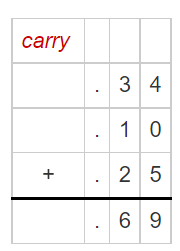Hence, from the above,
We can conclude that
The benchmark result for the given addition of numbers is: 0.75

Question 5.The given numbers are: 10.39, 4.28
Now,
We know that,
The benchmarks are: 0, 0.25, 0.75, and 1
So,Hence, from the above,
We can conclude that
The benchmark result for the given subtraction of numbers is: 6

Math Talk
Mathematical Processes

Describe the difference between an estimate and an exact answer.
An estimate is an answer to a problem that is close to the exact number, but not necessarily exact
An exact answer is an answer that is precise and need not be in a closed condition

Problem Solving

Use rounding or benchmarks to estimate.

Question 6.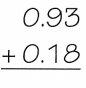The given numbers are: 0.93, 0.18
So,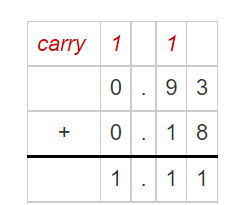Hence, from the above,
We can conclude that
The addition result of the given numbers by using the benchmark method is: 1

Question 7.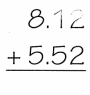The given numbers are: 8.12, 5.52
So,Hence, from the above,
We can conclude that
The addition result of the given numbers by using the rounding method is: 13.6

Question 8.The given numbers are: 9.75, 3.47
So,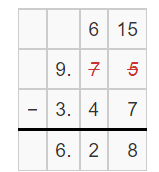Hence, from the above,
We can conclude that
The subtraction result of the given numbers by using the rounding method is: 6.3

Practice: Copy and Solve Use rounding or benchmarks to estimate.

Question 9.
12.83 + 16.24
The given numbers are: 12.83 and 16.24
So,Hence, from the above,
We can conclude that
The addition result of the given numbers by using the rounding method is: 29.1

Question 10.
$26.92 –$11.13
The given numbers are: 26,92 and 11.13
So,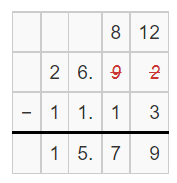Hence, from the above,
We can conclude that
The subtraction result of the given numbers by using the rounding method is: 15.8

Question 11.
9.41 + 3.82
The given numbers are: 9.41 and 3.82
So,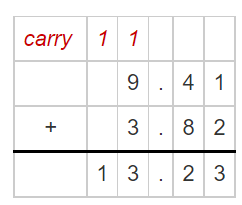Hence, from the above,
We can conclude that
The addition result of the given numbers by using the rounding method is: 13.23

H.O.T. Estimate to compare. Write < or >.

Question 12.
2.74 + 4.223.13 + 1.87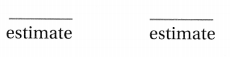The given addition expressions are: 2.74 + 4.22 and 3.13 + 1.87
So,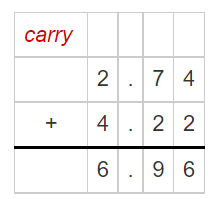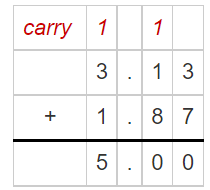So,
The estimated values of the given addition expressions respectively are: 7 and 5
Hence, from the above,
We can conclude that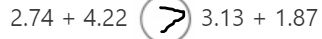Question 13.
6.25 – 2.399.79 – 3.84The given subtraction expressions are: 6.25 – 2.39 and 9.79 – 2.84
So,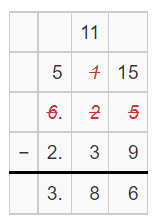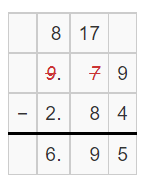So,
The estimated values of the given subtraction expressions respectively are: 4 and 7
Hence, from the above,
We can conclude that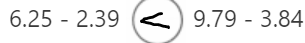Problem Solving

Use the table to solve 14. Show your work.Question 14.
Representations For the week of April 4, 1964, the Beatles had the top four songs. About how long would it take to listen to these four songs?
It is given that
For the week of April 4, 1964, the Beatles had the top four songs
Now,
The given table is:So,
The total duration that took to listen these four songs = 2.30 + 2.50 + 2.75 + 2.00
Now,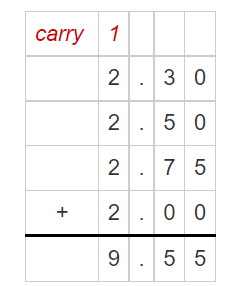Hence, from the above,
We can conclude that
The time that it took to listen to the given four songs is about 10 minutes

Use the table and estimation to solve 15-16.Question 15.
Representations Gina had a scrambled egg, an oat bran muffin, and a cup of low-fat milk for breakfast. About how many grams of protein did Gina have at breakfast?
It is given that
Gina had a scrambled egg, an oat bran muffin, and a cup of low-fat milk for breakfast
Now,
The given table is:Now,
From the given table,
We can observe that
The total amount of grams of protein did Gina have at breakfast = 6.75 + 3.99 + 8.22
Now,Hence, from the above,
We can conclude that
The total amount of grams of protein did Gina have at breakfast is about 19 grams

Question 16.
H.O.T. Multi-Step Pablo had a cup of shredded wheat cereal, a cup of low-fat milk, and one other item for breakfast. He had about 21 grams of protein. What was the third item Pablo had for breakfast?It is given that
Pablo had a cup of shredded wheat cereal, a cup of low-fat milk, and one other item for breakfast. He had about 21 grams of protein
Now,
The given table is:Now,
Let the amount of proteins present in the third item Pable had for breakfast be x grams
So,
According to the given information,
5.56 + 8.22 + x = 21
x = 21 – (5.56 + 8.22)
x = 21 – 13.78
Now,
By using the Long Subtraction,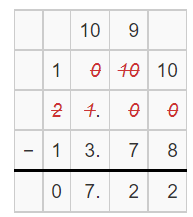Hence, from the above,
We can conclude that

Question 17.
Multi-Step Marco has room for 15 more minutes of songs on his mp3 player. He has three songs to upload with the following lengths: 5.15 minutes, 3.8 minutes, and 4.4 minutes. After uploading all three songs, how many minutes will he have left?
A. 3 minutes
B. 1 minute
C. 2 minutes
D. 0 minutes
It is given that
Marco has room for 15 more minutes of songs on his mp3 player. He has three songs to upload with the following lengths: 5.15 minutes, 3.8 minutes, and 4.4 minutes.
So,
The number of minutes Marco has left = 15 – (5.15 + 3.8 + 4.4)
= 15 – 13.35
= 1.65 minutes
≅ 2 minutes
Hence, from the above,
We can conclude that
The number of minutes Marco has left is: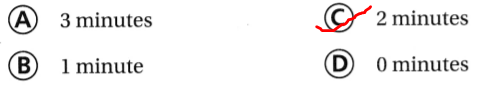Question 18.
Connect Geoff and Andrea want to combine their money to buy pizza. Geoff has $5.95. Andrea has$8.30. Which benchmarks should they use to estimate the money they have?
A. $6.00 +$8.25
B. $5.75 +$8.00
C. $6.00 +$8.50
D. $5.00 +$8.00
It is given that
Geoff and Andrea want to combine their money to buy pizza. Geoff has $5.95. Andrea has$8.30
So,
The total amount of money they should have = $5.95 +$8.30
Now,
By using the Benchmarks,
The estimated total amount of money they should have = $6 +$8.25
Hence, from the above,
We can conclude that
The benchmarks should they use to estimate the money they have is: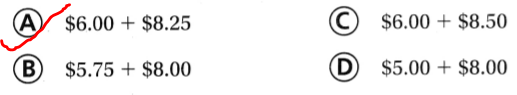Question 19.
Multi-Step Maiko wrote two songs. The first song was 2.9 minutes long. Then he added 0.4 minutes. The second song was 5.6 minutes long. Then he subtracted 1.1 minutes from it. Use benchmarks to estimate the length of each song.
A. first song: 2.5 minutes, second song: 4 minutes
B. first song: 3.5 minutes, second song: 4.5 minutes
C. first song: 3.5 minutes, second song: 7 minutes
D. first song: 2.5 minutes, second song: 5.5 minutes
It is given that
Maiko wrote two songs. The first song was 2.9 minutes long. Then he added 0.4 minutes. The second song was 5.6 minutes long. Then he subtracted 1.1 minutes from it.
Now,
According to the given information,
The duration of the first song = 2.9 + 0.4
= 3.3 minutes
The duration of the second song = 5.6 – 1.1
= 4.5 minutes
So,
By using the Benchmarks,
The duration of the first song is: 3.5 minutes
The duration of the second song is: 4.5 minutes
Hence, from the above,
We can conclude that
The estimation of the lengths of each song by using the benchmarks is: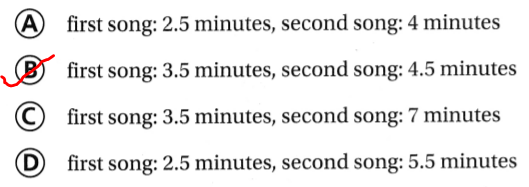Texas Test Prep

Question 20.
Fran bought sneakers for $54.26 and a shirt for$34.34. If Fran started with $100, how much money does she have left? A.$35
B. $20 C.$5
D. $80 Answer: It is given that Fran bought sneakers for$54.26 and a shirt for $34.34 and Fran started with$100
So,
The total amount of Fran has left = $100 – ($54.26 + 34.34)
= $100 –$88.60
= $11.40 Hence, from the above, We can conclude that The amount of money Fran has left is about: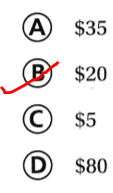### Texas Go Math Grade 5 Lesson 1.6 Homework and Practice Answer Key Use rounding or benchmarks to estimate. Question 1.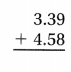Answer: The given numbers are: 3.39 and 4.58 So,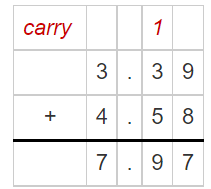Hence, from the above, We can conclude that The result of the given addition expression by rounded method is: 8 Question 2.Answer: The given numbers are: 6.77 and 3.23 So,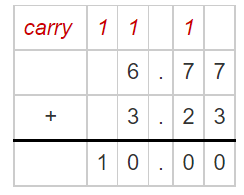Hence, from the above, We can conclude that The result of the given addition expression by benchmarks method is:10 Question 3.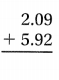Answer: The given numbers are: 2.09 and 5.92 So,Hence, from the above, We can conclude that The result of the given addition expression by benchmarks method is: 8 Question 4.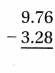Answer: The given numbers are: 9.76 and 3.28 So,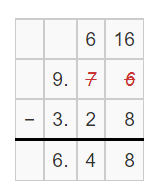Hence, from the above, We can conclude that The result of the given subtraction expression by rounding method is: 6.5 Question 5.Answer: The given numbers are: 7.36 and 0.83 So,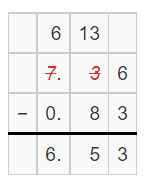Hence, from the above, We can conclude that The result of the given subtraction expression by rounding method is: 6.5 Question 6.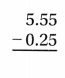Answer: The given numbers are: 5.55 and 0.25 So,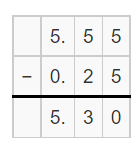Hence, from the above, We can conclude that The result of the given subtraction expression by rounding method is: 5.3 Estimate to compare. Write < or >. Question 7. 8.23 + 2.223.21 + 5.89Answer: The given addition expressions are: 8.23 + 2.22 and 3.21 + 5.89 Now, By using the Long Addition,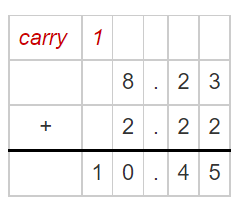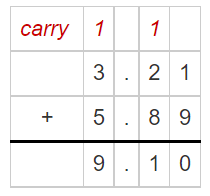So, The estimations of the given addition expressions are: 10.5 and 9.1 Hence, from the above, We can conclude thatQuestion 8. 7.32 – 3.769.23 – 4.28Answer: The given addition expressions are: 7.32 – 3.76 and 9.23 – 4.28 Now, By using the Long Subtraction,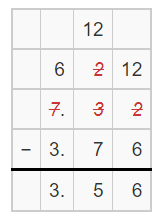So, The estimations of the given addition expressions are: 3.6 and 5 Hence, from the above, We can conclude that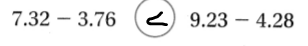Question 9.$18.25 + $5.50$10.75 + $15.00Answer: The given addition expressions are:$18.25 + $5.50 and$10.75 + $15.00 So,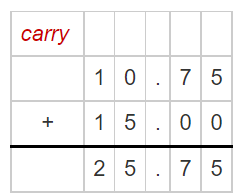So, The estimated values of the given addition expressions respectively are: 24 and 26 Hence, from the above, We can conclude thatQuestion 10. 6.2 – 4.87.23 – 5.08Answer: The given addition expressions are: 6.2 – 4.8 and 7.23 – 5.08 Now, By using the Long Subtraction,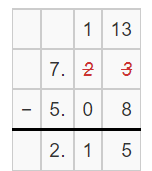So, The estimations of the given addition expressions are: 1.4 and 2.1 Hence, from the above, We can conclude thatProblem Solving Question 11. Li is running a race around the school track. He runs 0.327 kilometers during the first half of the race. What is the distance that he travels in the first half of the race, rounded to the nearest hundredth? Answer: It is given that Li is running a race around the school track. He runs 0.327 kilometers during the first half of the race. Now, When we rounded to the nearest hundredth, The distance that Li traveled around the school track will become: 0.33 kilometers Hence, from the above, We can conclude that The distance that Li travels in the first half of the race, rounded to the nearest hundredth is: 0.33 kilometers Question 12. A video lasts 6.44 minutes. About how long does the video last, rounded to the nearest minute? Answer: It is given that A video lasts 6.44 minutes Now, The duration of video when rounded to the nearest tenth is: 6 minutes Hence, from the above, We can conclude that The video lasts about 6 minutes when rounded to the nearest minute Lesson Check Fill in the bubble completely to show your answer. Question 13. Dylan wants to buy a book for$16.95 and a magazine for $4.50. About how much will he need to buy the items without having to round down to the nearest benchmark? A.$15
B. $30 C.$25
D. $20 Answer: It is given that Dylan wants to buy a book for$16.95 and a magazine for $4.50 So, The amount of money Dylan will need =$16.95 + $4.50 Now, By using the Long Addition,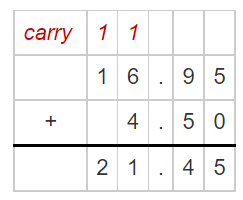SO, The amount of money Dylan will need, when rounded to the nearest benchmark is:$20
Hence, from the above,
We can conclude that
The amount of money will Dylan need to buy the items without having to round down to the nearest benchmark is:Question 14.
Sasha downloads a song that is 4.32 minutes long and one that is 8.28 minutes long. About how many minutes will it take all of her songs to play if she already has 13.8 minutes of songs?
A. 15 minutes
B. 30 minutes
C. 20 minutes
D. 26 minutes
It is given that
Sasha downloads a song that is 4.32 minutes long and one that is 8.28 minutes long
Now,
Let x be the total time
So,
According to the given information,
x = 13.8 + (4.32 + 8.28)
x  = 13.8 + 12.6
x = 13.8 + 12.6
x = 26.4 minutes
x ≅ 26 minutes
Hence, from the above,
We can conclude that
The number of minutes will it take all of sasha’s songs to play is:Question 15.
Mike spends $23.40 on a present for his mom and$18.95 on a present for his dad. If Mike started with $75, how much money does he have left? A.$30
B. $50 C.$20
D. $40 Answer: It is given that Mike spends$23.40 on a present for his mom and a $18.95 on a present for his dad. If Mike started with$75
So,
The amount of money does Mike spend = $75 – ($23.40 + $18.95) =$75 – $42.35 =$32.65
≅ $30 Hence, from the above, We can conclude that The amount of money does Mike had left is:Question 16. Damelle and Raj want to combine their money to buy a soccer ball. Danielle has$7.95. Raj has $6.35. Which benchmarks should they use to estimate the money they have? A.$7.75 + $6.50 B.$8.00 + $6.25 C.$7.50 + $6.50 D.$6.00 + $6.50 Answer: It is given that Damelle and Raj want to combine their money to buy a soccer ball. Danielle has$7.95. Raj has $6.35. So, The amount of money they have =$7.95 + $6.35 So, The amount of money they have when rounded to the nearest benchmark =$7.75 + \$6.50
Hence, from the above,
We can conclude that
The benchmarks should they use to estimate the money they have is: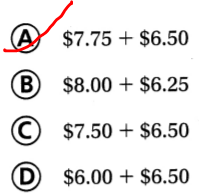Question 17.
Multi-Step Jesse, Max, and Kalel each ate 0.32 of a pizza pie. Which number shows the best estimate of how much of the pie they ate together?
A. 1.0
B. 0.5
C. 0.25
D. 0.10
It is given that
Jesse, Max, and Kalel each ate 0.32 of a pizza pie
So,
The amount of pizza pie they ate = 0.32 + 0.32 + 0.32
= 0.64 + 0.32
= 0.96
≅ 1
Hence, from the above,
We can conclude that
The number that shows the best estimate of the amount of pie they ate together is: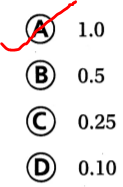Question 18.
Multi-Step George is giving two speeches at school. The first was 3.4 minutes long. Then he added 0.9 minutes to the speech. The second speech was 4.1 minutes long. Then he subtracted 1.4 minutes from it. Use benchmarks to estimate the length of each speech.
A. first speech: 4.0 minutes; second speech: 3.5 minutes
B. first speech: 4.0 minutes; second speech: 3 minutes
C. first speech: 4.5 minutes; second speech: 2.5 minutes
D. first speech: 4.5 minutes; second speech: 3.5 minutes
It is given that
George is giving two speeches at school. The first was 3.4 minutes long. Then he added 0.9 minutes to the speech. The second speech was 4.1 minutes long. Then he subtracted 1.4 minutes from it
So,
The duration of the first speech = 3.4 + 0.9
= 4.3 minutes
The duration of the second speech = 4.1 – 1.4
= 2.7 seconds
Now,
By using the Benchmarks,
The duration of the first speech is: 4 minutes
The duration of the second speech is: 3 minutes
Hence, from the above,
We can conclude that
By uing Benchmarks, the length of each speech is:Scroll to Top